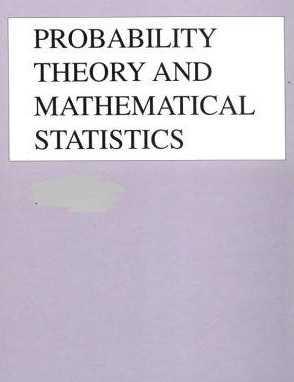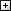Processing ......FreeComputerBooks.com Free Computer, Mathematics, Technical Books and Lecture Notes, etc.

Probability Theory and Mathematical Statistics
To track Un-filtered Flights at any place in the world, click here.
• Title Probability Theory and Mathematical Statistics
• Author(s) Prasanna Sahoo
• Publisher: University of Louisville (2013)
• Hardcover/Paperback N/A
• eBook PDF
• Language: English
• ISBN-10/ASIN: N/A
• ISBN-13: N/ABook Description

This book is an introduction to probability and mathematical statistics intended for students already having some elementary mathematical background.

This book is both a tutorial and a textbook. This book presents an introduction to probability and mathematical statistics and it is intended for students already having some elementary mathematical background. It is intended for a one-year junior or senior level undergraduate or beginning graduate level course in probability theory and mathematical statistics.

The book contains more material than normally would be taught in a one-year course. This should give the teacher flexibility with respect to the selection of the content and level at which the book is to be used. This book is based on over 15 years of lectures in senior level calculus based courses in probability theory and mathematical statistics at the University of Louisville.

Probability theory and mathematical statistics are dicult subjects both for students to comprehend and teachers to explain. Despite the publication of a great many textbooks in this field, each one intended to provide an improvement over the previous textbooks, this subject is still dicult to comprehend. A good set of examples makes these subjects easy to understand.

For this reason alone the author has included more than 350 completely worked out examples and over 165 illustrations. He gives a rigorous treatment of the fundamentals of probability and statistics using mostly calculus. He has given great attention to the clarity of the presentation of the materials. In the text, theoretical results are presented as theorems, propositions or lemmas, of which as a rule rigorous proofs are given. For the few exceptions to this rule references are given to indicate where details can be found. This book contains over 450 problems of varying degrees of diculty to help students master their problem solving skill.

Reviews, Ratings, and Recommendations: Related Book Categories: Read and Download Links:Similar Books:All CategoriesRecent BooksIT Research LibraryMiscellaneous BooksComputer LanguagesComputer ScienceData Science/DatabasesElectronic EngineeringJava and Java EE (J2EE)Linux and UnixMathematicsMicrosoft and .NETMobile ComputingNetworking and CommunicationsSoftware EngineeringSpecial TopicsWeb Programming